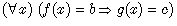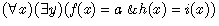Chapter 2

An Outline of the Mizar Language

The Mizar language is used for describing mathematical demonstrations by using computers. Because we normally follow a method of writing demonstrations when we write a Mizar article, we have to write with the Mizar language. From now, we abbreviate Mizar language to Mizar.
In Mizar, we use general ASCII code. Letters that are usually used are numbers, English alphabet, symbols. Also, for mathematical symbols, there are ones that use similar things or combine general ASCII characters .
Also, in Mizar, we have to keep in mind that capital and lower-case letters of the English alphabet are distinguished. Therefore, when we write MS-DOS names of articles and file names into an article, we always have to use capital letters. In this case, we write within 8 capital English letters, numbers, and, _, '.
As we use such words as 'therefore', 'theorem', 'definition', and 'end of demonstration' when writing mathematical demonstrations; in Mizar, we also use corresponding words to describe. These words become reserved words. As a Mizar system, the logic of &, or, not, concept of =, and predicate logic of All, Exist are prepared from the beginning. Moreover, we press on with our demonstrations by phrasing definitions and theorems of functor, predicate, and others that are registered in the Mizar library; however, their basic is written in HIDDEN.ABS. The materials that are written here is accepted as axioms without demonstrations. We structured the Mizar library by phrasing those and leading different theorems. When users write new articles, they describe by phrasing logic that are already prepared in the system from the beginning or the numerous theorems that are created based upon things such as TARSKI.
When users define functor function and others, they first register those into the vocabulary file and then define them in an article. In this case, we cannot define a functor that has exactly the same name with a reserved word. The method for defining specifically is explained in chapter 6. Also, please look at the table of Mizar reserved words in the appendix.
We will now discuss the logical formula and predicate logic that are prepared in the Mizar system. We can use the basic logical formula such as &, or, not, implies, and iff. For example, the following can be written.

A　&　B
A = B　&　B = C impliesA = C
A & ( B or C ) iff A & B or A & C

& is used for the logical formula. For between the formulas, we use and. Also, implies and iff indicate 'then' and if only if 'equivalence'.
For the predicate logic, we can use all symbols and exist symbols like the following.

for x st f[x] = b holds g[x] = c;

For the writing method of the normal predicate logic, this indicates the following formula.This means for an optional x, if f(x) = b, then g(x) = c. Therefore, it means that for all x, g(x) = c that satisfies f (x) = b will be formed.
Further, f[x], g[x] are the predicates that include x and it does not mean that this kind of writing method is prepared in the system.
Next, if we write the following,

ex x st f[x] =b & g[x] =c ;

it means the formula below.

(∃x)(f (x) =b and g (x) =c )

It means that x that becomes g (x) = c (when f(x) =b) exists. Also, we can mix all and existent symbols.

for x ex y st f[x] = a & h [x] = i [y]

The above formula indicates the following:We are allowed to use parentheses as an option. Even when parentheses are not needed, we can use them for better understanding.
In addition, there are the MS-DOS version and the Linux version in the Mizar system. Furthermore, although it is essentially the Linux version, there is a Mizar system which can be used for freedom from a Web page.